Function Repository Resource:

# QuantumDiscreteStateToZXDiagram

Convert a pure quantum discrete state into a ZX-diagram

Contributed by: Jonathan Gorard
 ResourceFunction["QuantumDiscreteStateToZXDiagram"][QuantumDiscreteState[…]] converts the specified pure QuantumDiscreteState into an equivalent ZXDiagramObject.

## Details

If ResourceFunction["QuantumDiscreteStateToZXDiagram"] succeeds in converting the specified QuantumDiscreteState into a ZX-diagram, it will return a ZXDiagramObject expression.
ResourceFunction["QuantumDiscreteStateToZXDiagram"] works by partially tracing out each qubit in the specified QuantumDiscreteState individually. Every qubit of the general form {1,α} is converted into a Z-spider generator in the resulting ZXDiagramObject, and every qubit of the general form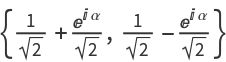is converted into an X-spider generator in the resulting ZXDiagramObject. Any qubits that are not found to be equivalent to one of these two forms are omitted.
Whenever the specified QuantumDiscreteState cannot be converted into an equivalent MakeZXDiagramObject via the above procedure, ResourceFunction["QuantumDiscreteStateToZXDiagram"] will return Indeterminate.
Since it is representing a pure QuantumDiscreteState, every generator/spider produced within the resulting ZXDiagramObject will have an input arity of 0 and an output arity of 1.
In the resulting ZXDiagramObject, the following properties are supported:
 "LabeledGraph" graph form of the ZX-diagram with phases labeled "UnlabeledGraph" graph form of the ZX-diagram without phases labeled "OperatorForm" ZX-diagram represented as a tensor product of generators "ListForm" ZX-diagram represented as a list of generators "MatrixForm" ZX-diagram represented as an explicit linear map on qubits "ZSpiders" list of Z-spiders in the ZX-diagram "XSpiders" list of X-spiders in the ZX-diagram "HadamardGates" list of Hadamard gates in the ZX-diagram "Diamonds" list of black diamonds in the ZX-diagram "Wires" list of wires in the ZX-diagram "ZSpiderCount" number of Z-spiders in the ZX-diagram "XSpiderCount" number of X-spiders in the ZX-diagram "HadamardGateCount" number of Hadamard gates in the ZX-diagram "DiamondCount" number of black diamonds in the ZX-diagram "WireCount" number of wires in the ZX-diagram
ResourceFunction["QuantumDiscreteStateToZXDiagram"] names the ZX generators in the resulting ZXDiagramObject in the conventional way, naming Z-spiders as z1,z2,; X-spiders as x1,x2,; black diamonds as d1,d2,; outputs as o1,o2,; etc.

## Examples

### Basic Examples (2)

Convert a single-qubit pure discrete quantum state into a single-spider ZX-diagram:

 In:=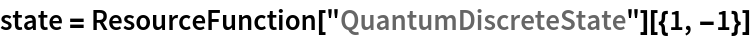Out=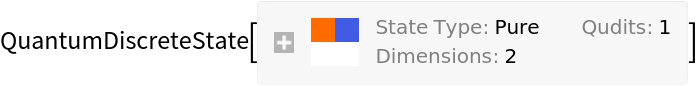In:=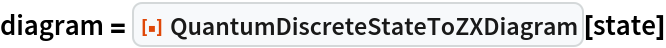Out=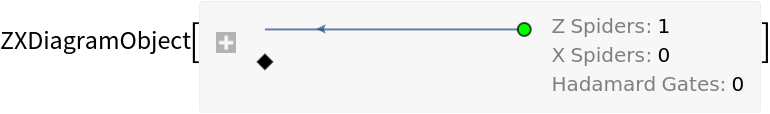In:=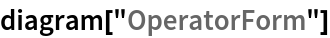Out=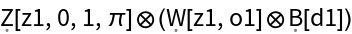In:=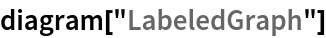Out=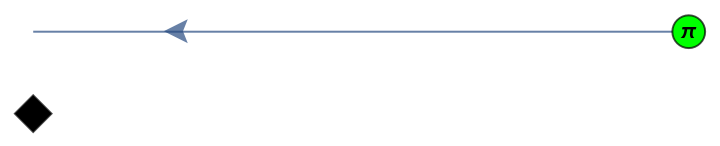Convert a different single-qubit pure discrete quantum state into a single-spider ZX-diagram:

 In:=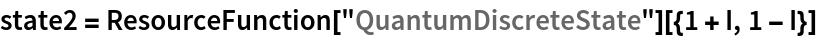Out=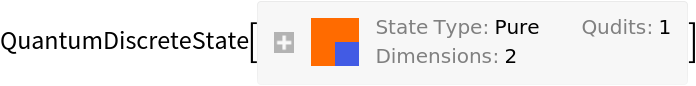In:=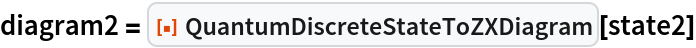Out=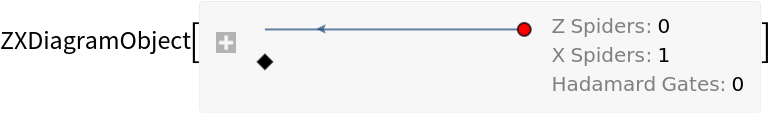In:=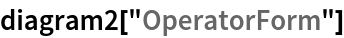Out=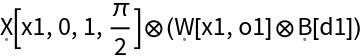In:=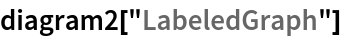Out=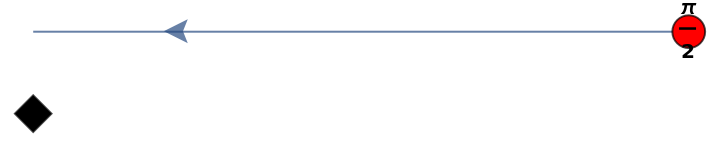Convert a more complicated three-qubit pure discrete quantum state into a three-spider ZX-diagram:

 In:=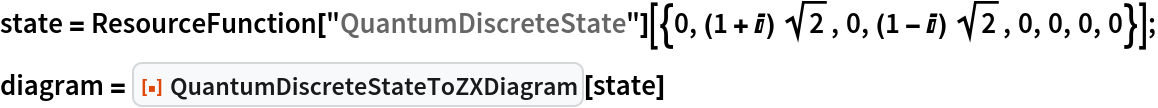Out=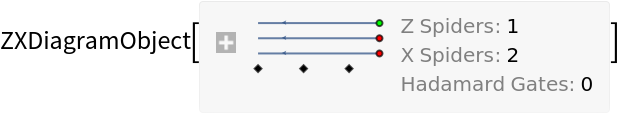In:=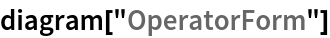Out=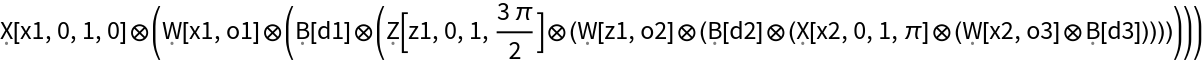In:=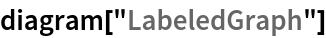Out=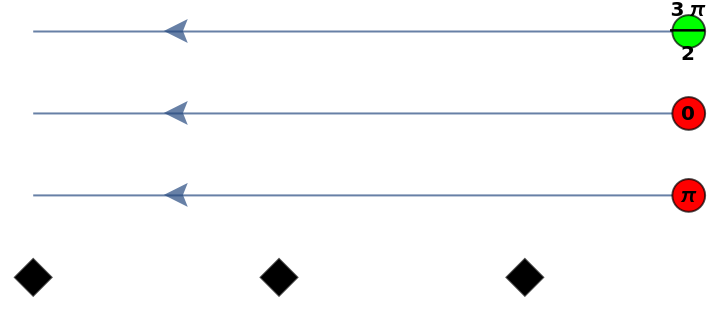Convert a different four-qubit pure discrete quantum state into a four-spider ZX-diagram:

 In:=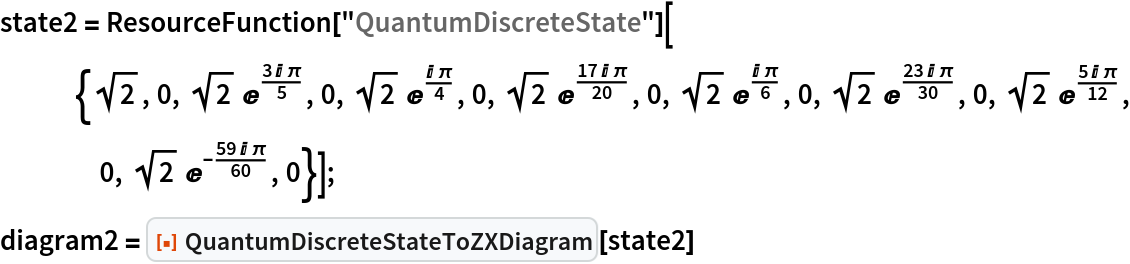Out=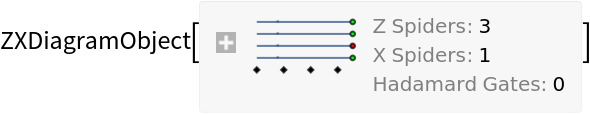In:=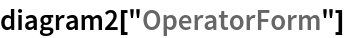Out=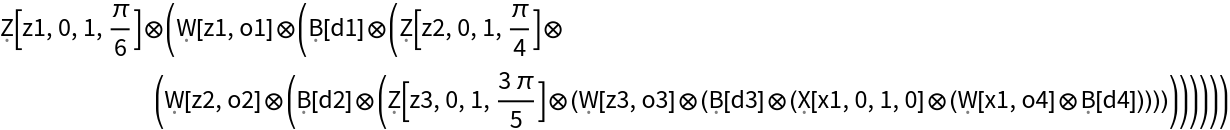In:=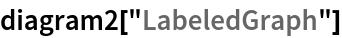Out=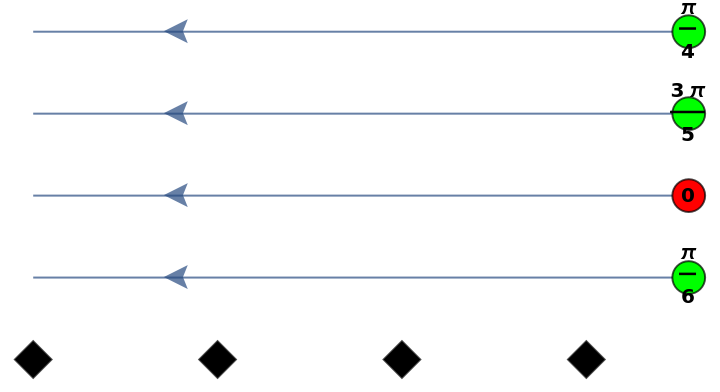### Scope (1)

If the specified QuantumDiscreteState object cannot be expressed as a ZX-diagram, QuantumDiscreteStateToZXDiagram will return Indeterminate:

 In:=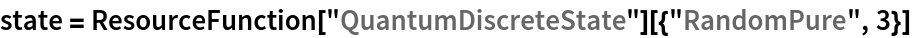Out=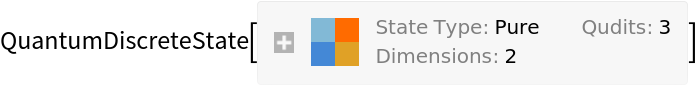In:=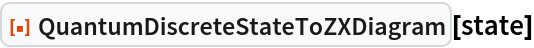Out=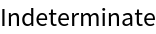Jonathan Gorard

## Version History

• 1.0.0 – 26 April 2021